Your browser does not support the IFRAME tag.Home MonkeyNotes Printable Notes Digital Library Study Guides Study Smart Parents Tips College Planning Test Prep Fun Zone Help / FAQ How to Cite New Title Request

 8.5 Arc Length ( Rectification ) The method of finding the length of the arc of a curve is called the rectification. For arc length, the function and its derivative must both be continuous on the closed interval. If y = f (x) and f (x) are continuous on [ a, b ], then the arc length ( L ) of f (x) on [ a,b ] is given bySimilarly for, x = f (y) and f (y) are continuous on [ a,b ], then the arc length (L) of f (y) on [ a,b ] is given byExample 29 Find the length of the arc of f (x) = x3/2 on [ 0, 5 ]. Solution :both are continuous on [ 0, 5 ]. then length of arc of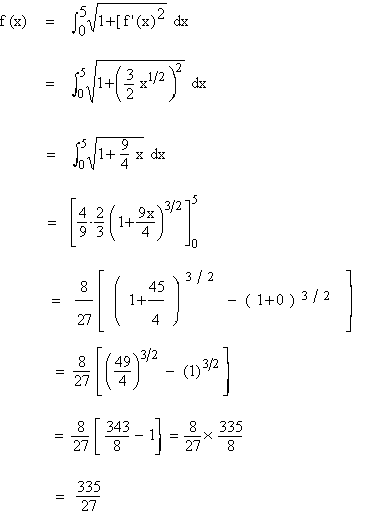Example 30 Find the length of the arc of the parabola y2 = 12x cut off by the Latus rectum. Solution : y2 = 12 x ® Parabola. Comparing with y2 = 4 ax, we see that 4 a = 12 \ a = 3 \ AS = SL = 6 \ co-ordinates of L are (3,6)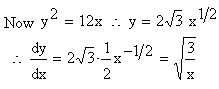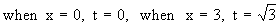Example 31 Find the length of the curve y2 = (2 x - 1 )3, cut off by the line x = 4. Solution : y2 = (2 x - 1 )3 is a curve (i) symmetrical about the x-axis (ii) not passing through (0,0) (iii) it cuts the x-axis, where y = 0 \ x = ½ \ It's vertex is at ( ½ , 0) (iv) It does not cut the y-axis as, taking x = 0 we get y = ± i (v) No asymptotes. (vi) (2x - 1)3 positive \ y2 ³ 0 \ x ³ ½ \ The curve lies in only 1st and 4th quadrants Now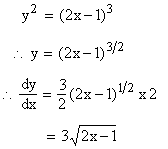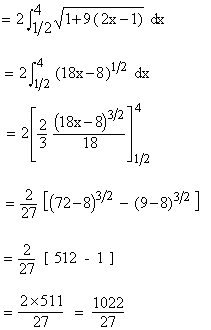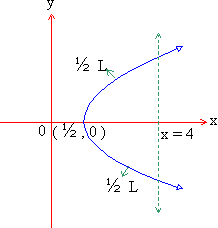Index 8.1 Introduction8.2 Area 8.3 Volumes 8.4 Mean Value 8.5 Arc Length(Rectification) Chapter 1